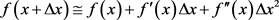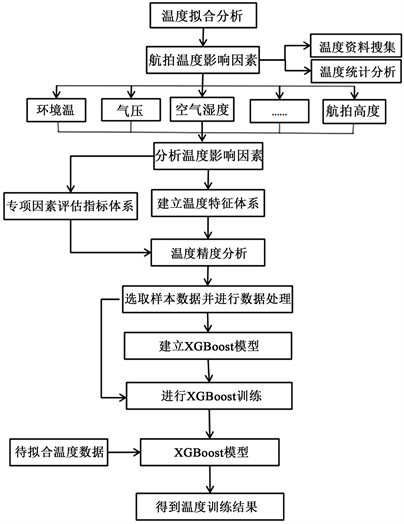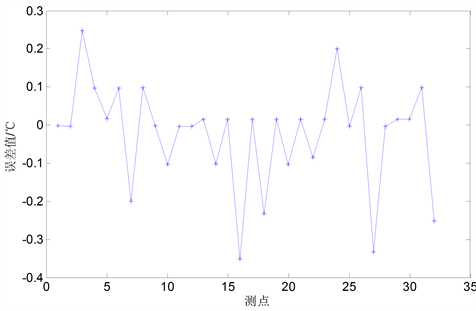# 基于XGBoost的无人机测温误差分析Analysis of Temperature Measurement Error of Unmanned Aerial Vehicle Based on XGBoost

DOI: 10.12677/GST.2019.74022, PDF, 下载: 463  浏览: 1,073  国家自然科学基金支持

Abstract: Under the influence of the flying height of the UAV, the roll angle, the pitch angle, the direction an-gle, the speed and so on, the temperature values of the UAV have some error. The factors affecting the infrared temperature measurement accuracy are taken into consideration, and the XGBoost into the machine learning is adopted. The temperature prediction and the error analysis of tem-perature measurement are used to predict the ideal temperature value, and the key factors af-fecting the error of temperature measurement are obtained.

1. 引言

2. XGBoost原理

2.1. XGBoost原理

$Obj\left(\theta \right)=L\left(\theta \right)+\Omega \left(\theta \right)$ (1)

$Ob{j}_{m}={\sum }_{i=1}^{n}l\left(\left({y}_{i},{\stackrel{^}{y}}_{i}^{m-1}\right)+{f}_{m}\left({x}_{i}\right)\right)+\Omega \left({f}_{m}\right)$ (2)(3)

${g}_{i}={\partial }_{\stackrel{^}{y}m-1}l\left({y}_{i},{\stackrel{^}{y}}^{m-1}\right)$ (4)

${h}_{i}={\partial }_{\stackrel{^}{y}m-1}^{2}l\left({y}_{i},{\stackrel{^}{y}}^{m-1}\right)$ (5)

2.2. XGBoost优势

3. 航拍试验

3.1. 测温实验

3.2. 数据预处理

1) 数据筛选

2) 确定空间分辨率

3) 图像定位

4) 畸变校正

5) 船测温度与航空温度对比提取

6) 气象参数的提取

3.3. 测温误差分析

4. XGBoost提高测温精度

4.1. XGBoost拟合温度实验

1) 特征选择Table 1. The main features of modeling

2) 温度预测流程Figure 1. Flow chart of temperature prediction

3) 参数的优化

XGBoost模型存在大量可以调节的参数，例如eta、max_depth、nround、subsample、alpha、lambda、min_child_weight等；根据多次实验发现，XGBoost准确率虽然较高，但仍然有较高的提升空间，故而可以将参数逐个进行优化，每优化一个参数便可以提升一定的预测精度。

max_depth = 5

learning_rate = 0.1

n_estimators = 75

silent = True

objective = 'reg:linear'

gamma = 0

min_child_weight = 1

max_delta_step = 0

subsample = 0.85

colsample_bytree = 0.72

colsample_bylevel = 1

reg_alpha = 0

reg_lambda = 1

scale_pos_weight = 1

seed = 1440

4) 实验结果Figure 2. Temperature fitting comparison chartFigure 3. Temperature fitting comparison chartTable 2. Comparison and analysis table of prediction temperature accuracy

4.2. 测温误差分析

4.2.1. 影响因素重要度分析

plot_importance(xgb_boost, importance_type = 'weight')

pyplot.show()

print(xgb_boost.feature_importances_)Figure 4. Importance of influencing factors

4.2.2. 姿态角的影响Figure 5. Temperature prediction error graph

4.2.3. 风速的影响Figure 6. Temperature prediction error graph

4.2.4. 湿度、气温的影响

1) 气温与湿度共同影响Figure 7. Temperature prediction error graph

1) 气温、湿度单独作用下的影响Figure 8. Temperature and humidity temperature error comparison chart

2) 气温、湿度影响误差分析

5. 结束语

NOTES

*通讯作者。

  贺佳惠, 梁春利, 李名松. 核电站近岸温度场航空热红外遥感测量数据处理研究[J]. 国土资源遥感, 2010(3): 51-53.  何园. 遥感技术在核电站温排水影响范围监测中的应用[J]. 科技创新与应用, 2016(18): 71.  大连航天北斗科技有限公司. 辽宁红沿河核电站无人机航空遥感海水测温技术方案[R]. 2016: 7.  苏佳伟, 石俊生, 汪炜穑. 距离对红外成像仪测温精度影响及提高精度的实验研究[J]. 红外技术, 2013, 35(9): 587-590.  张健, 杨立, 刘慧开. 环境高温物体对红外热像仪测温误差的影响[J]. 红外技术, 2005(5): 419-422.  官上洪, 王毕艺, 赵万利, 闫秀生. 红外热像仪测温精度分析[J]. 光电技术应用, 2012, 27(3): 85-88.  陈超帅, 王世勇. 大疆无人机目标红外辐射特性测量及温度反演[J]. 光电工程, 2017, 44(4): 427-434+464.  伊晓东, 孙鹏. 基于小波分析的神经网络在遥感测温数据拟合中的应用[J]. 测绘与空间地理信息, 2017, 40(5): 8-10+13+16.  Chen, T. and Guestrin, C. (2016) XGBoost: A Scalable Tree Boosting System. 22nd ACM SIGKDD In-ternational Conference on Knowledge Discovery and Data Mining, San Francisco, 13-17 August 2016, 785-794. https://doi.org/10.1145/2939672.2939785